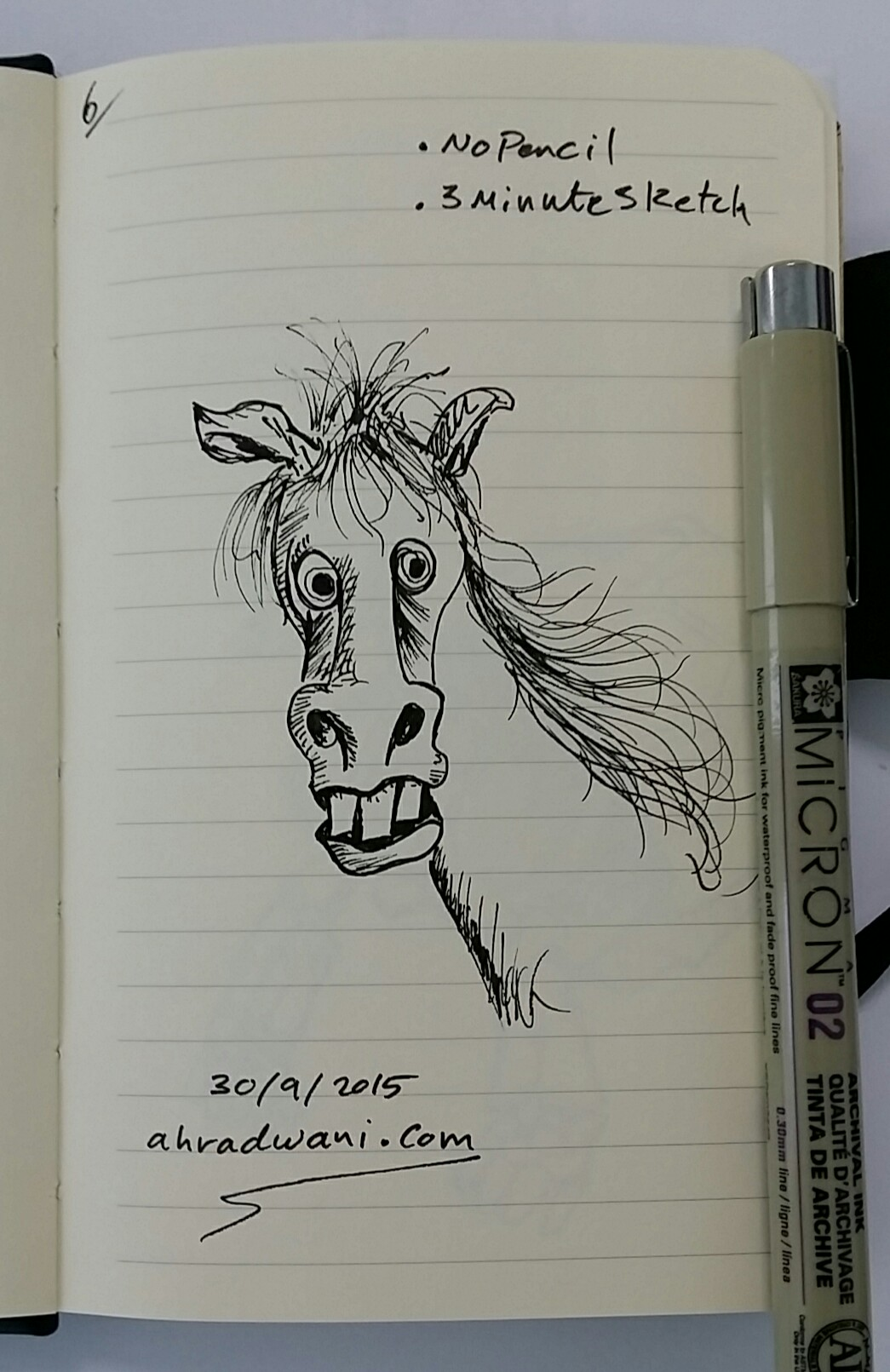## Python: My Fake Data Generator P-2

Learning : Python: Functions, Procedures and documentation
Subject: About fake data P-2: (Fake ID)

Before we start i’d like to mention that with our last fcolor() function we write some comments in the first part of the function between three double quote(“””), and if we load the function and call help() as help(fcolor()) we will get that information on the python console as a help as in screen shot.

In this post we will write a function to generate a fake ID number, for ID’s there could be several styles, sometime we just want a random number without any meaning; just X number of random digits. Most of the time we need this number to be mean-full based on certain rules. For example, in Banks they may use some digits that indicate the branch. In sport club, they may include the date … and so-on.

Here we will write a function called key_generator(), the function will take two arguments (dig, s) dig is your key digits number, s is the style, if s = d then the first 6 digits of the key will be the date as ddmmyy + random digits, and if s = anything else or s not passed then the key will be as default (just x-digits). Let’s see the code.

First the summary or say information about the function:

```def key_generator(dig, s = 'n'):
"""
### Date: 8/12/2019, By: Ali Radwani ###
Summary:
This function will generate x-digit key randomly.
If the argument s = 'd' or 'D' then the key is two part, first (6) digits
are date as ddmmyy then x-digit random numbers.

If the argument s anything else than ['d','D'] or no argument passes, then the key
is random numbers without any meaning.

The numbers will randomly be selected in range of (10 to 99).

import: random, datetime

Argument: int: dig: The number of digits for the key.
str: s  : The key style (with date or just random numbers)

return: int: the_key
"""
```

Now, if the user pass s=’d’ then part of the key will be the current date, to do this we will call the datetime function in python and split it into dd,mm,yy. Here is the key_generator() function.

```def key_generator(dig, s = 'n'):
"""
### Date: 8/12/2019, By: Ali Radwani ###
Summary:
This function will generate x-digit key randomly.
If the argument s = 'd' or 'D' then the key is two part, first (6) digits
are date as ddmmyy then x-digit random numbers.

If the argument s anything else than ['d','D'] or no argument passes, then the key
is random numbers without any meaning.

The numbers will randomly be selected in range of (10 to 99).

import: random, datetime

Argument: int: dig: The number of digits for the key.
str: s  : The key style (with date or just random numbers)

return: int: the_key
"""
the_key=''
if s in ['d','D'] :
d = str(datetime.date.today())
dd = d[8:10]
mm = d[5:7]
yy = d[2:4]
the_key = dd + mm + yy
for x in range (dig):
the_key = the_key + str( random.randint(10,99))
return int(the_key[:(dig + 6)])

else :
for x in range (dig):
the_key = the_key + str( random.randint(10,99))

return int(the_key[:dig])
```

In next Fake Data function we will try to write one to generate the date. It will be published on next Sunday.

:: Fake Function List ::

 Function Name Description Color To return a random color code in RGB or Hex. Date To return a random date. Mobile To return a mobile number. Country To return a random country name. City To return a random City name. ID To return X random dig as ID. Time To return random time.

 DoneFollow me on Twitter..

## Python: Smallest Multiple

Python: Smallest multiple
Problem 5 @ projecteuler
Completed on: Thu, 4 Jul 2019, 22:30

Here I am quoting form ProjectEuler site:”

2520 is the smallest number that can be divided by each of the numbers from 1 to 10 without any remainder. What is the smallest positive number that is evenly divisible by all of the numbers from 1 to 20?”

So to solve this simple task all we need to loop through numbers and divide it by a list of (1,20) if yes return True otherwise return False and got to another number.
and so we done..

The Code:

codes hereFollow me on Twitter..

## My Sketchbook

I am sketching to enhance my skill, here is one of sketches from my sketchbook using pencil as a guide-line and ink-pen to finish the drawing.Click to Enlarge

::SketchBook::
Using: Ink-Pen
Date: 30.9.2015
SketchBook: SketchBook # 5Follow me on Twitter..Ali,

## New Section to to Blog

I create a new section under Menu> Pages and I call it My Sketches I am posting some of my sketches using the Sketchbook apps, I am using this app on my iPhone, iPad and on my Galaxy Tab, on my iPhone and because of the screen protection cover I can’t use the pen to draw so I am using my finger; very difficult to draw.

Here are Two of my Sketches… [Click to Enlarge]